• 2022-01-02 14:45:08

## 如何评估？

简单线性回归 的 目标是找到 a 和 b 使得 ∑ i = 1 m ( y t r a i n ( i ) − a x t r a i n ( i ) − b ) 2 \sum^m_{i=1} (y_{train}^{(i)} - ax_{train}^{(i)} - b )^2 尽可能小。

这个式子也可以表示为 ∑ i = 1 m ( y t r a i n ( i ) − y ^ t r a i n ( i ) ) 2 \sum^m_{i=1} ( y_{train}^{(i)} - \hat{y}_{train}^{(i)} )^2

训练完成后得到 y ^ t r a i n ( i ) = a x t e s t ( i ) + b \hat{y}_{train}^{(i)} = ax_{test}^{(i)} + b

### MSE

所以衡量回归问题的标准可以是（m 是测试数据集的个数）：

1 m ∑ i = 1 m ( y t e s t ( i ) − y ^ t e s t ( i ) ) 2 \frac{1}{m} \sum^m_{i=1} ( y_{test}^{(i)} - \hat{y}_{test}^{(i)} )^2

这个标准也叫 均方误差MSE(Mean Squared Error)；

### RMSE

MSE 的问题是，得到的数值是 量纲的平方，所以需要开根号，得到的公式称为 均方根误差 RMSE(Root Mean Squared Error )

1 m ∑ i = 1 m ( y t e s t ( i ) − y ^ t e s t ( i ) ) 2 = M S E t e s t \sqrt{ \frac{1}{m} \sum^m_{i=1} ( y_{test}^{(i)} - \hat{y}_{test}^{(i)} )^2 } = \sqrt{ MSE_{test} }

MSE 和 RMSE 区别不大，主要看对量纲的敏感性。

线性回归还有另一个评测方法：平均绝对误差 MAE (Mean Absolute Error)

1 m ∑ i = 1 m ∣ y t e s t ( i ) − y ^ t e s t ( i ) ∣ \frac{1}{m} \sum^m_{i=1} | y_{test}^{(i)} - \hat{y}_{test}^{(i)} |

绝对值不是处处可导，所以没有用在损失函数中；但绝对值完全可以用来评价。
评价一个算法的标准 和 训练模型使用的最优化函数的标准 是可以完全不一致的。

### RMSE & MAE

RMSE 得到的值一般比 MAE 大；
RMSE 有平方操作，会放大样本中预测结果和真实结果 较大误差 的趋势。MAE 没有这个趋势。

所以一般来讲，让 RMSE 尽量小（比 MAE ）意义更大，因为这意味着 样本的错误中，最大的错误值 相应的比较小。

## R Square

分类问题的评估 比较简单，主要看 分类的准确度，在 0–1 之间进行取值。
但 RMSE 和 MAE 没有这种性质，R Square （也可读作 R方）这个方法解决了这个问题。
R 方比较重要，使用比较广泛。

公式：
R 2 = 1 − S S r e s i d u a l S S t o t a l = 1 − ∑ i ( y ^ ( i ) − y ( i ) ) 2 ∑ i y ‾ ( i ) − y ( i ) ) 2 R^2 = 1 - \frac{ SS_{residual} }{ SS_{total} } = 1 - \frac{ \sum_i ( \hat{y}^{(i)} - y^{(i)} )^2 }{ \sum_i \overline{y}^{(i)} - y^{(i)} )^2 }

= 1 − ( ∑ i ( y ^ ( i ) − y ( i ) ) 2 ) / m ( ∑ i y ‾ ( i ) − y ( i ) ) 2 ) / m = 1 − M S E ( y ^ , y ) V a r ( y ) = 1 - \frac{ (\sum_i ( \hat{y}^{(i)} - y^{(i)} )^2 )/m }{ (\sum_i \overline{y}^{(i)} - y^{(i)} )^2)/m } = 1 - \frac{MSE( \hat{y}, y )}{ Var(y) }

residual：Residual Sum of Squares
total：Total Sum of Squares

分子 ∑ i ( y ^ ( i ) − y ( i ) ) 2 \sum_i ( \hat{y}^{(i)} - y^{(i)} )^2 代表使用我们的模型来预测，产生的错误；

分母 ∑ i y ‾ ( i ) − y ( i ) ) 2 \sum_i \overline{y}^{(i)} - y^{(i)} )^2 ，也可以看做一个模型，代表使用 $y = \overline{y}$ 预测产生的错误。这是个很朴素的预测结果，在统计学领域称为 基准模型（Baseline Model）；这个模型预测的错误率是比较高的。

使用 1 来减去这个分数，相当于 我们的模型没有产生错误的比率。

### R 方特性

• R^2<= 1
• R^2 越大越好。当我们的预测模型不犯任何错误时，R^2 取得最大值1；
• 当我们的模型等于基准模型时，R^2为0，是最差的情况；
• 如果R^2< 0，说明我们学习到的模型还不如基准模型。此时，很有可能我们的数据不存在任何线性关系。

## 示例：SKLearn 预测boston 房价数据并评估

### 读取、查看数据特征

import numpy as np
import matplotlib.pyplot as plt
from sklearn import datasets

boston.keys()
# dict_keys(['data', 'target', 'feature_names', 'DESCR', 'filename'])

boston.DESCR
# 有 13 个特征

boston.feature_names
# array(['CRIM', 'ZN', 'INDUS', 'CHAS', 'NOX', 'RM', 'AGE', 'DIS', 'RAD', 'TAX', 'PTRATIO', 'B', 'LSTAT'], dtype='<U7')

boston.data
'''
array([[6.3200e-03, 1.8000e+01, 2.3100e+00, ..., 1.5300e+01, 3.9690e+02,
4.9800e+00],
...,
[6.0760e-02, 0.0000e+00, 1.1930e+01, ..., 2.1000e+01, 3.9690e+02, 5.6400e+00],
[4.7410e-02, 0.0000e+00, 1.1930e+01, ..., 2.1000e+01, 3.9690e+02, 7.8800e+00]])
'''

x = boston.data[:,5] # 取第五列数据，使用房间数量这个特征
x.shape # (506,)

y = boston.target
y # array([24. , 21.6, 34.7, 33.4, 36.2, 28.7, 22.9, 27.1, 16.5, 18.9, 15. ,  20.6, 21.2, 19.1, 20.6, 15.2,  7. ,  8.1,...20.6, 23.9, 22. , 11.9])


### 先做一个简单线性回归

## 看看数据分布

plt.scatter(x, y)

# 最上方分布的点，贴近最大值，可能是异常点，采集的时候计量的限制值。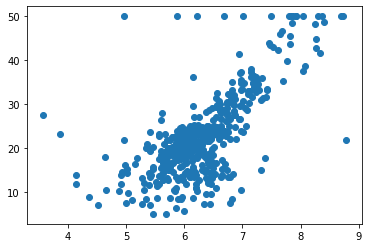### 去除异常值

# 确认有最大值
np.max(y) # 50.0

# 删除最大值的点
x = x[y < 50.0]
y = y[y < 50.0]

plt.scatter(x, y)
# 这里就没有上述的边缘点了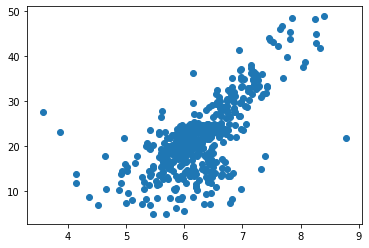### 训练数据

from sklearn.model_selection import train_test_split

x_train, x_test, y_train, y_test = train_test_split( x, y, test_size=0.2, random_state=42)

x_train.shape, x_test.shape # ((392,), (98,))

from sklearn.linear_model import LinearRegression

lr = LinearRegression()

x_train = x_train.reshape(-1, 1)
y_train = y_train.reshape(-1, 1)

lr.fit(x_train, y_train)
# LinearRegression(copy_X=True, fit_intercept=True, n_jobs=None, normalize=False)

# 斜率，权重列表
lr.coef_   # array([[8.24882251]])

# 截距 bias值
lr.intercept_  # array([-29.6721626])

plt.scatter(x_train, y_train)
plt.plot(x_train, lr.predict(x_train), color='r')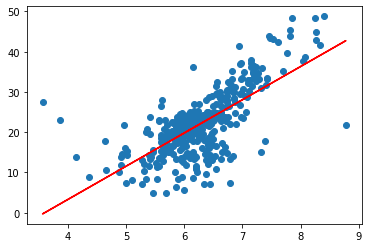### 预测并评估

# 预测
y_predict = lr.predict(x_test.reshape(-1,1))
y_test = y_test.reshape(-1,1)

## 计算 MSE

mse_test = np.sum((y_predict - y_test)**2) / len(y_test)
mse_test # 31.763084786207326

## RMSE
rmse_test = np.sqrt(mse_test)
rmse_test # 5.6358748022119265

# MAE
mae_test = np.sum( np.abs(y_predict - y_test)) / len(y_test)
mae_test # 3.9395943900511363

# R Square
rsquare = 1 - mean_squared_error(y_test, y_predict)/ np.var(y_test)
rsquare  # 0.37823504497807936


### 使用 sklearn.metrics 中的方法进行评估

from sklearn.metrics import mean_squared_error, mean_absolute_error, r2_score

# mse
mean_squared_error(y_test, y_predict)  # 31.763084786207326

# mae
mean_absolute_error(y_test, y_predict) # 3.9395943900511363

# R Square
r2_score(y_test, y_predict) # 0.37823504497807936



## PyTorch 中的损失函数

### mse

import torch.nn as nn
import torch

mse = nn.MSELoss()

# t1 tensor([-0.5326, -2.1040, -0.0849,  0.0078, -0.3299], requires_grad=True)
# t2 tensor([-0.3427,  0.5773, -0.8011, -0.6496, -0.9095], requires_grad=True)

mse(t1, t2)



### 二分类 bce


# t1 tensor([ 0.3398,  0.8650, -1.2867, -1.4845,  0.6145], requires_grad=True)

# 分类标签概率值
t1s = torch.sigmoid(t1)  # 求 sigmoid 函数，转化为 （0，1） 之间的概率
# t1s tensor([0.5841, 0.7037, 0.2164, 0.1847, 0.6490], grad_fn=<SigmoidBackward>)

# 目标数据值；随机生成 0，1 的整数序列，并转化为浮点数
t2 = torch.randint(0, 2, (5, )).float()
# t2 tensor([1., 0., 1., 1., 0.])

bce = nn.BCELoss()
bce(t1s, t2) # 计算二分类的交叉熵；接收的两个参数都必须是浮点数

# 对数（Logits）交叉损失函数；可以直接省略 sigmoid 计算部分；自动在函数内部添加 sigmoid 激活函数；
# 在训练时，使用这个函数可以增加计算数值的稳定性。
bce_logits = nn.BCEWithLogitsLoss()
bce_logits(t1, t2) # 与上方结果一致


### 多分类

N = 10 # 分类数目
t2 = torch.randint(0, N, (5, ))
# t2  tensor([7, 5, 3, 2, 5])

t1s = nn.functional.log_softmax(t1, -1)

# 负对数似然函数。
# 根据预测值（经过 softmax 的计算和对数计算） 和目标值（使用独热编码）计算这两个值 按照一一对应的乘积，然后对乘积求和，并取负值。
# 使用它之前，必须先计算 softmax 函数取对数的结果。
n11 = nn.NLLLoss()
n11(t1s, t2)

# 可以避免 LogSoftmax 计算
# 在损失函数中整合 Softmax 输出概率，以及对概率取对数输出损失函数
ce = nn.CrossEntropyLoss()
ce(t1, t2)线性回归 机器学习
更多相关内容
• RMSE评价算法，RMSE一般指均方根误差。均方根误差亦称标准误差。 RMSE（A，B）：A为原图，B为类比图
• 几种ESPRIT算法（doa估计）性能的对比分析（RMSE对比）
• 如何轻松计算RMSE，添加Keyey = 7367462 激活，示例： r = RMSE（cell2mat（数据），ceil（预测），7367462）；matlab
•matlab
• 此函数参考原始信号计算信号的 RMSE（均方根误差）。 可以为 1-D/2-D/3-D 信号计算 RMSEmatlab
• 在keras的loss库中添加rmse方法，成功使模型能正常使用rmse方法。在metrics.py文件上添加rmse方法后可以使用model.compile(loss='mse'，weighted_metrics=['rmse'])方法，可以在其他loss的条件下观测rmse的变化
• 几种music-DOA估计算法的性能比较（RMSE对比）
• Matlab程序，用于求取MSE均方误差，可直接在程序中调用，MSE用来检测模型的预测值与真实值之间的偏差，值越大表示预测效果越差
• 今天小编就为大家分享一篇python之MSE、MAE、RMSE的使用，具有很好的参考价值，希望对大家有所帮助。一起跟随小编过来看看吧
• [Mse, Dse, stdL1, stdL2, alfa1]=RM_vec2rmse(uN, vN, uM, vM); ％ 输入% uN 是向量 N 的东向分量% vN 是向量 N 的北向分量％uM是向量M的向东分量% vM 是向量 M 的北向分量 ％ 输出% Mse 是系统误差向量的模块...matlab
• MAE、MSE、R-Square、MAPE和RMSE 以上是对于预测问题的评价指标。 1.平均绝对误差（Mean Absolute Error, MAE） 误差越大，该值越大。 2.均方误差（Mean Squared Error, MSE） 误差越大，该值越大。 SSE(和方差)与...
• 这是一个利用所包含的函数来计算 8 个图像指标（偏差、相关系数、DIV、熵、ERGAS、Q、RASE 和 RMSE）的程序。 该程序的目的是为用户快速、轻松和方便地生成结果（参见输出）。 最初，它的目的是在高光谱和多光谱...matlab
• 利用Python计算Excel表里多个sheet的均方根误差（RMSE）。前提是在已经知晓你的Excel表中有多少个Sheet，使用for循环，快速计算每个sheet的均方根误差。
• 几种ESPRIT算法（doa估计）性能的对比分析（RMSE对比）
• 含psnr,rmse, ergas, sam, uiqi,ssim,DD,CCS，matlab版图片评估指标，适用与高光谱图像，多波段图像 Computes a number of quality indices from the remote sensing literature, namely the RMSE, ERGAS, SAM and ...
• 详细介绍了基于均匀线阵型的波达方向估计算法，线性旋转不变性子空间方法tls-esprit，介绍了RMSE变化的情况，具有可操作性。
• matlab计算rmse代码无味卡尔曼滤波器项目 背景 在本项目中，您将使用 C++ 编写 Unscented Kalman 滤波器程序，通过嘈杂的激光雷达和雷达测量来估计感兴趣的非线性移动对象的状态。 卡尔曼滤波器是一种强大的方法来...
• 基于协同过滤的推荐系统，关于求RMSE的部分源码，简单易懂，易操作！学习python,对机器学习，推荐系统方面感兴趣的可以参考。协同过滤
• 卡尔曼滤波UKF实现2D目标跟踪 代码肯定能运行且有结果，可开发性强， 如果有问题可联系WX:ZB...仿真结果：二维跟踪轨迹，位置RMSE，速度RMSE（均方根误差） 各维度跟踪轨迹，跟踪误差误差，各个维度跟踪误差）。
• 该代码可用于包括DOA估计算法中，进行自适应APES算法与MUSIC算法的均方根误差对比的计算，自变量为信噪比变化，其中包含数个子程序，有已成形的仿真图。
• 对Vondrak滤波的准则函数进行了探讨，提出了一种新的评价标准，即用滤波值的均方根误差与平滑度的线性组合的平方根δ作为平滑因子质量好坏的评价标准。模拟算例证实了此评价标准的可行性。
• 推荐系统 利用MovieLens数据，Pearson相似度，分别基于User和Item构建一个简单的kNN推荐系统，并进行RMSE评价
• 你一定要知道的预测评价指标MSE、RMSE、MAE、MAPE、SMAPE！！！

💖作者简介：大家好，我是车神哥，府学路18号的车神🥇
📝个人主页：应无所住而生其心的博客_府学路18号车神_CSDN博客
🎉点赞评论收藏 == 养成习惯（一键三连）😋
📖本系列主要以学习Go语言打怪升级为标准，实现自我能力的提升为目标⚡
⚡希望大家多多支持🤗~一起加油 😁

### 预测评价指标

最近论文在写关于极限学习机ELM的相关内容，在机器学习中有很重要的一点就是评级指标，这是判断你的算法性能很重要的、很有必要的一个评判标准，下面我们就一起来看看有哪些评价指标吧！~

# 背景

机器学习中，一般是对输出值，具体也就是对预测值 Y ^ \hat Y 和真实值 Y Y 进行评价，利用以下的评价指标来表现预测和真实之间的差距，误差越小说明效果越好，性能越好！~

这里我们假设：
Y ^ = { y ^ 1 , y ^ 2 , . . . , y ^ n } − − 预 测 值 \hat{Y}=\{\hat{y}_1,\hat{y}_2,...,\hat{y}_n\}--预测值

Y = { y 1 , y 2 , . . . , y n } − − 预 测 值 {Y}=\{{y}_1,{y}_2,...,{y}_n\}--预测值

# 均方误差（MSE）

均方误差（Mean Square Error，MSE）,反映估计量与被估计量之间差异程度的一种度量。设t是根据子样确定的总体参数θ的一个估计量，(θ-t)2的数学期望，称为估计量t的均方误差。它等于σ2+b2，其中σ2与b分别是t的方差与偏倚。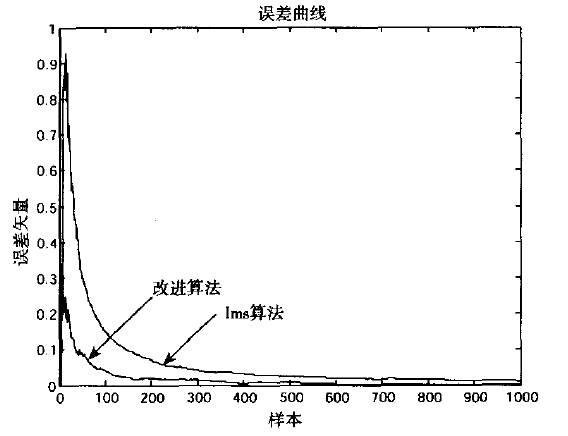MSE

MSE计算公式：
M S E = 1 n ∑ i = 1 n ( y ^ i − y i ) 2 {MSE}=\frac{1}{n} \sum_{i=1}^{n}\left(\hat{y}_{i}-y_{i}\right)^{2}

解释：

范围[0,+∞)，当预测值与真实值完全吻合时等于0，即完美模型；误差越大，该值越大。
总而言之，值越小，机器学习网络模型越精确，相反，则越差。

# 均方根误差（RMSE）

均方根误差（Root Mean Square Error，RMSE），从名称来看，我们都能猜得到是什么意思。多了一个根，这个“根”的意思顾名思义，就只是加了个根号。均方根误差是预测值与真实值偏差的平方与观测次数n比值的平方根，在实际测量中，观测次数n总是有限的，真值只能用最可信赖（最佳）值来代替。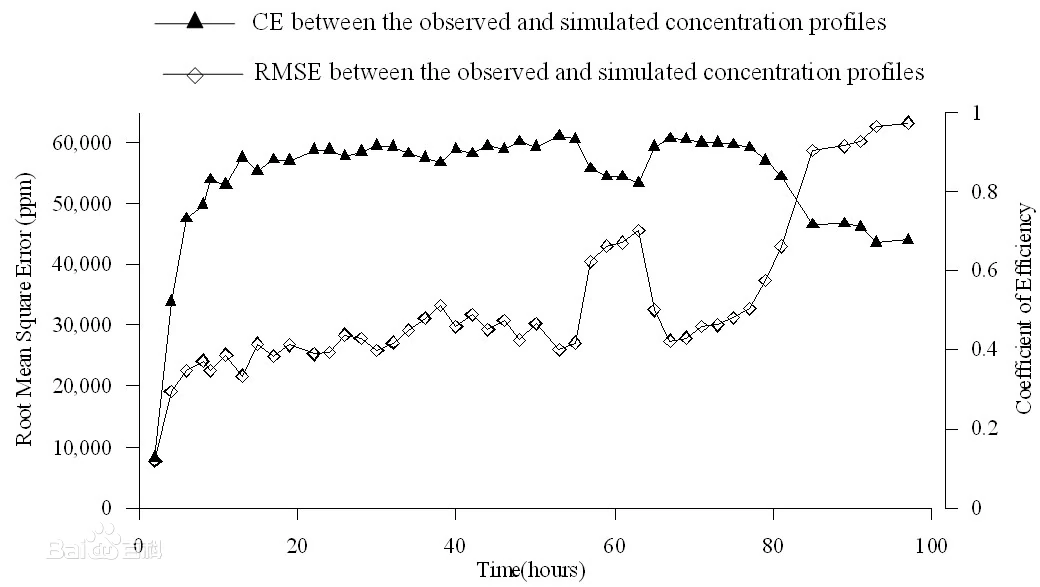RMSE的计算公式：
R M S E = 1 n ∑ i = 1 n ( y ^ i − y i ) 2 RMSE=\sqrt{\frac{1}{n} \sum_{i=1}^{n}\left(\hat{y}_{i}-y_{i}\right)^{2}}

解释：

它的计算方法是先平方、再平均、然后开方。均方根误差是用来衡量观测值同真值之间的偏差。和MSE同理，当我们的预测值和真实值之间的差距越小，模型精度越高；相反，则越低。

# 平均绝对误差（MAE）

平均绝对误差（Mean Absolute Error，MAE）,绝对偏差平均值即平均偏差，指各次测量值的绝对偏差绝对值的平均值。平均绝对误差可以避免误差相互抵消的问题，因而可以准确反映实际预测误差的大小。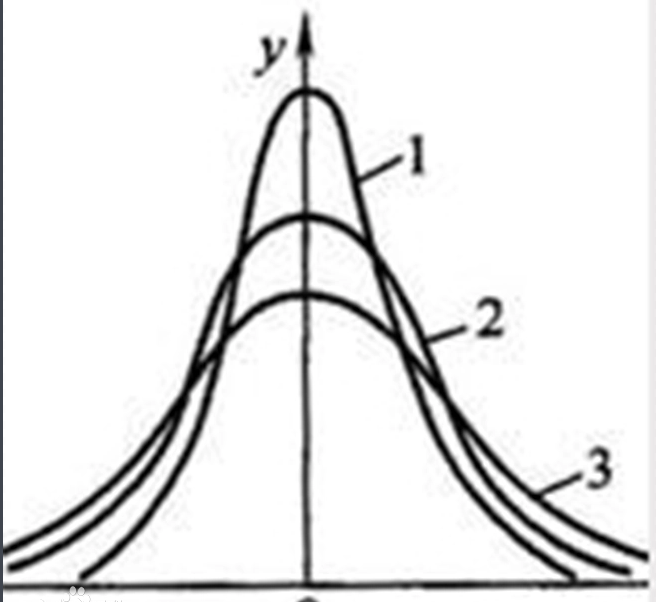MAE

MAE计算公式：
M A E = 1 n ∑ i = 1 n ∣ y ^ i − y i ∣ M A E=\frac{1}{n} \sum_{i=1}^{n}\left|\hat{y}_{i}-y_{i}\right|

解释：

范围[0,+∞)，和MSE、RMSE类似，当预测值和真实值的差距越小，则模型越好；相反则越差。

# 平均绝对百分比误差（MAPE）

平均绝对百分比误差（Mean Absolute Percentage Error，MAPE），平均绝对百分比误差之所以可以描述准确度是因为平均绝对百分比误差本身常用于衡量预测准确性的统计指标,如时间序列的预测。

计算公式：
M A P E = 100 % n ∑ i = 1 n ∣ y ^ i − y i y i ∣ M A P E=\frac{100 \%}{n} \sum_{i=1}^{n}\left|\frac{\hat{y}_{i}-y_{i}}{y_{i}}\right|

解释：

和上面的MAE相比，在预测值和真实值的差值下面分母多了一项，除以真实值。
范围[0,+∞)，MAPE 为0%表示完美模型，MAPE 大于 100 %则表示劣质模型。

需要注意的一点！！！

当真实值有数据等于0时，存在分母0除问题，该公式不可用！

# 对称平均绝对百分比误差（SMAPE）

对称平均绝对百分比误差（Symmetric Mean Absolute Percentage Error，SMAPE）

SMAPE计算公式为：
S M A P E = 100 % n ∑ i = 1 n ∣ y ^ i − y i ∣ ( ∣ y ^ i ∣ + ∣ y i ∣ ) / 2 S M A P E=\frac{100 \%}{n} \sum_{i=1}^{n} \frac{\left|\hat{y}_{i}-y_{i}\right|}{\left(\left|\hat{y}_{i}\right|+\left|y_{i}\right|\right) / 2}

解释：

与MAPE相比，加了对称，其实就是将分母变为了真实值和预测值的中值。和MAPE的用法一样，范围[0,+∞)，MAPE 为0%表示完美模型，MAPE 大于 100 %则表示劣质模型。

同样，值得注意的一点！！！

当真实值有数据等于0，而预测值也等于0时，存在分母0除问题，该公式不可用！

这里也给出一下Python代码：

#!/usr/bin/env python
# -*- coding: utf-8 -*-
# @Time    : 2021/12/21 15:05
# @Author  : 府学路18号车神
# @Email   ：yurz_control@163.com
# @File    : Evaluation_index.py

import numpy as np
from sklearn import metrics

# 将sklearn的也封装一下吧
# MSE
def mse(y_true, y_pred):

res_mse = metrics.mean_squared_error(y_true, y_pred)

return res_mse
# RMSE
def rmse(y_true, y_pred):

res_rmse = np.sqrt(metrics.mean_squared_error(y_true, y_pred))

return res_rmse

# MAE
def mae(y_true, y_pred):

res_mae = metrics.mean_absolute_error(y_true, y_pred)

return res_mae

# sklearn的库中没有MAPE和SMAPE，下面根据公式给出算法实现
# MAPE
def mape(y_true, y_pred):

res_mape = np.mean(np.abs((y_pred - y_true) / y_true)) * 100

return res_mape

# SMAPE
def smape(y_true, y_pred):

res_smape = 2.0 * np.mean(np.abs(y_pred - y_true) / (np.abs(y_pred) + np.abs(y_true))) * 100

return res_smape

# main
if __name__=='__main__':

# 由于没有用模型，这里就随机出几个值来测试下吧

y_true = np.random.random(10)
print(y_true)
y_pred = np.random.random(10)
print(y_pred)

# MSE
print(mse(y_true, y_pred))
# RMSE
print(rmse(y_true, y_pred))
# MAE
print(mae(y_true, y_pred))
# MAPE
print(mape(y_true, y_pred)) # 得到的值直接看成百分比即可
# SMAPE
print(smape(y_true, y_pred)) # 得到的值直接看成百分比即可❤坚持读Paper，坚持做笔记，坚持学习，坚持刷力扣LeetCode❤！！！
坚持刷题！！！打天梯！！！
To Be No.1

⚡⚡

创作不易⚡，过路能❤关注收藏点个赞三连就最好不过了

ღ( ´･ᴗ･` )

只是相谈就会开心起来，沉浸在温柔的眼神当中，竭尽全力的思念，悄悄地奉献。

展开全文机器学习 人工智能 python 预测评价指标
• 预测问题评价指标： 预 MAE、MSE、R-Square、MAPE和 RMSE MAE、MSE、R-Square、MAPE和RMSE 以上是对于预测问题的评价指标。 1.平均绝对误差（Mean Absolute Error, MAE） 误差越大，该值越大。 2.均方误差（Mean ......